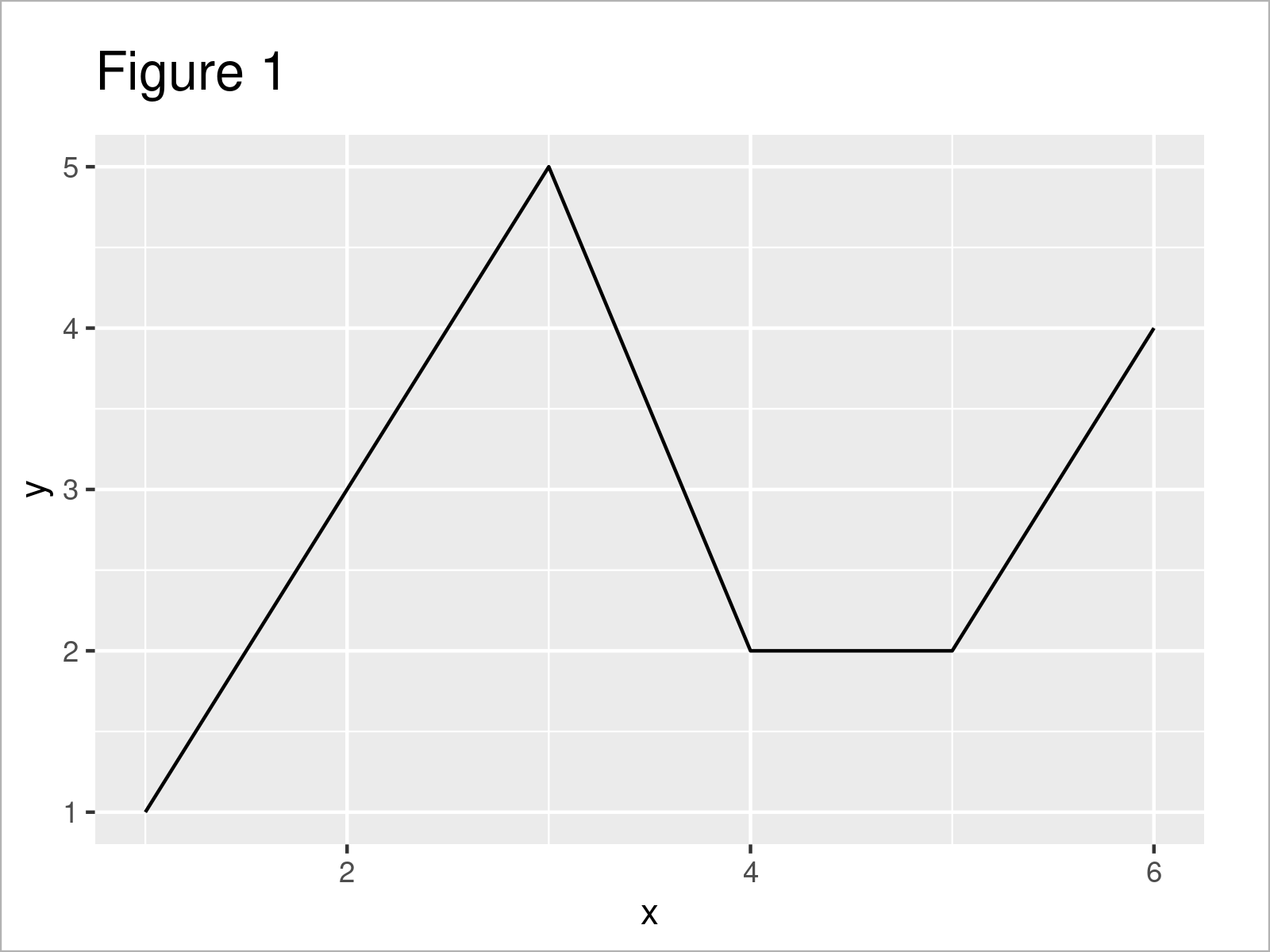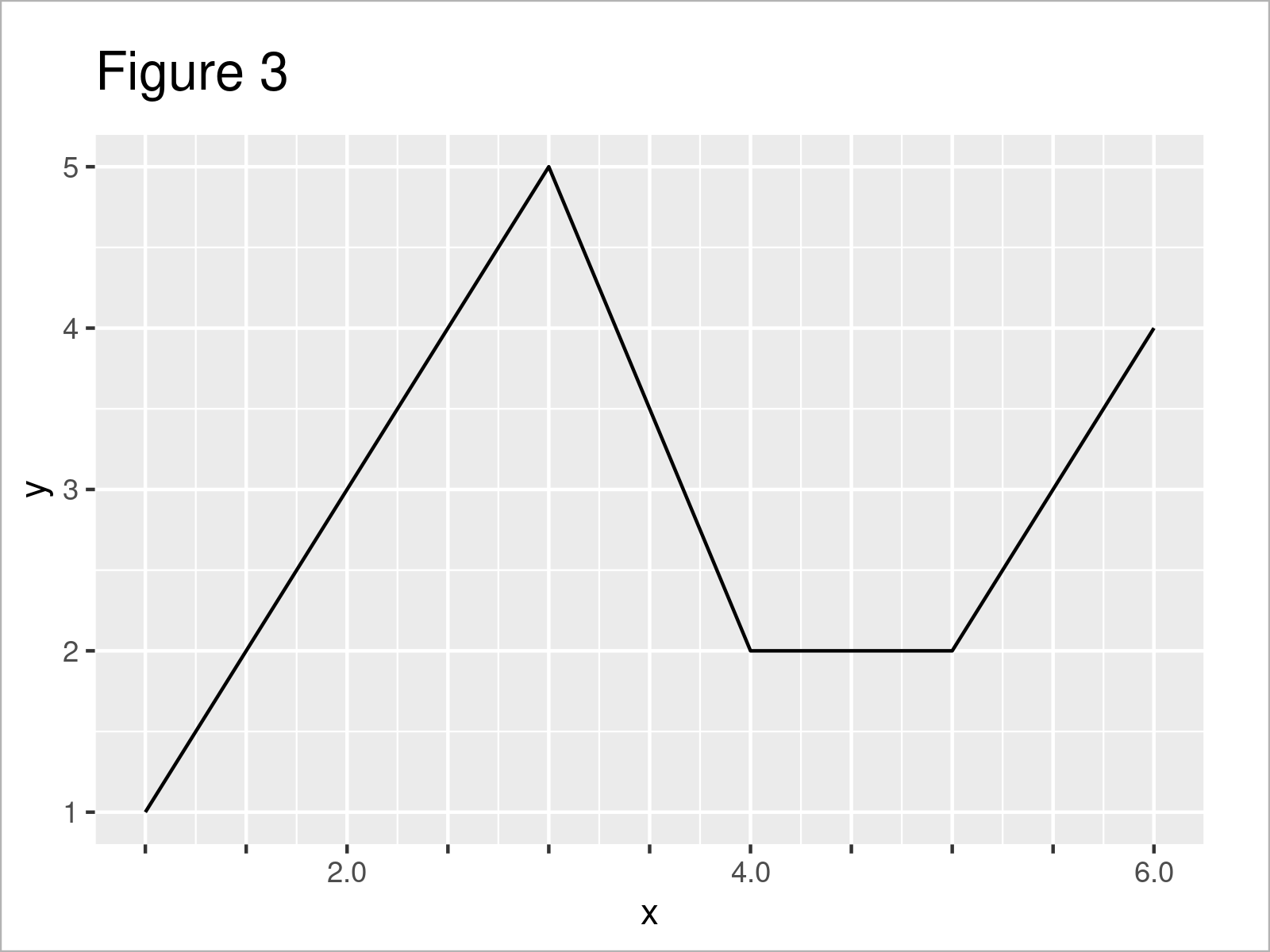# Add Minor Tick Marks without Labels to ggplot2 Plot in R (Example)

This tutorial shows how to draw additional minor tick marks without labels in a ggplot2 plot in the R programming language.

Let’s dive right into the example…

## Example Data, Packages & Basic Graph

The data below will be used as a basis for this R programming tutorial.

```data <- data.frame(x = 1:6,                # Create example data frame
y = c(1, 3, 5, 2, 2, 4))
data                                       # Print example data frame```Table 1 shows that our example data contains six rows and two variables.

In order to draw our data using the ggplot2 package, we also have to install and load ggplot2:

```install.packages("ggplot2")                # Install & load ggplot2 package
library("ggplot2")```

Next, we can plot our data.

```ggp <- ggplot(data, aes(x, y)) +           # Create ggplot2 plot with default axes
geom_line()
ggp                                        # Draw ggplot2 plot with default axes```As shown in Figure 1, we have managed to create a new ggplot2 plot with default specifications for the minor and major tick marks, as well as for the corresponding grid lines.

## Example: Draw ggplot2 Plot with Additional Axis Tick Marks without Labels

The code below demonstrates how to add additional axis tick marks without labels to a ggplot2 graphic.

As a first step, we have to specify the breaks (i.e. the tick marks) that we want to use in our plot. For this, we can use the seq() function as shown below:

```my_breaks <- seq(1, 6, by = 0.5)           # Create sequence for new x-axis
#   1.0 1.5 2.0 2.5 3.0 3.5 4.0 4.5 5.0 5.5 6.0
my_breaks                                  # Print sequence for new x-axis```

We may now use this sequence to create a ggplot2 plot with modified axis ticks and grid lines:

```ggp +                                      # Draw ggplot2 plot with new x-axis
scale_x_continuous(breaks = my_breaks)```Figure 2 visualizes the output of the previous R syntax: A ggplot2 plot with additional axis ticks on the x-axis.

However, at this point all the new axis ticks have labels. Let’s change that!

To drop some of the axis labels, we have to create another vector with the same length as our breaks vector. This vector needs to contain the labels that we want to show as well as a blank character for the labels we do not want to show:

```my_labels <- sprintf("%.1f", my_breaks)    # Specify blanks for unnecessary labels
#   ""    ""    "2.0" ""    ""    ""    "4.0" ""    ""    ""    "6.0"
my_labels[c(1, 2, 4, 5, 6, 8, 9, 10)] <- ""
my_labels                                  # Print updated labels```

Finally, we can draw our plot with additional axis tick marks:

```ggp +                                      # Additional minor axis ticks without labels
scale_x_continuous(breaks = my_breaks,
labels = my_labels)```As shown in Figure 3, we have created a ggplot2 graph with additional axis tick marks, but no additional labels.

Note that we could use the same logic to add further axis tick marks on the y-axis.

## Video, Further Resources & Summary

I have recently published a video on my YouTube channel, which shows the examples of the present article. You can find the video below:

Please accept YouTube cookies to play this video. By accepting you will be accessing content from YouTube, a service provided by an external third party.If you accept this notice, your choice will be saved and the page will refresh.

Furthermore, you could have a look at the other articles on my homepage: